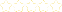+
Graph of Tangent

# Graph of Tangent

You may know what the Graph of Tangent looks like, but why does it take on the shape it does? To answer this question you need to have an understanding of Trigonometric Identities and how tangent is related to both the Sine Wave and the Cosine Wave.With this knowledge you will also understand the relationship between asymptotes and the tangent function.

Learn this and go on to Graph of Cotangent
2 Tutorials That Teach Graph of Tangent

#### Graph of Tangent

Author: Ronald
Views: 4183
Rating:(2)

#### Graph of Tangent

Author: Todd
Views: 2096
Rating:(1)